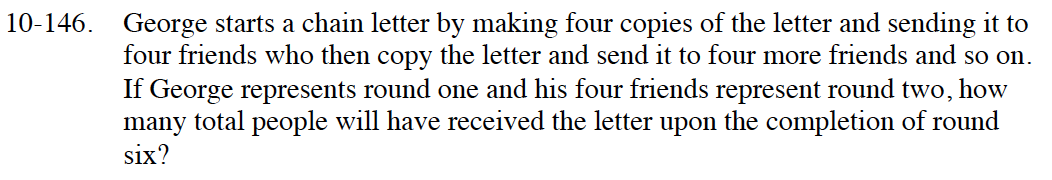### Home > INT3 > Chapter 10 > Lesson 10.3.1 > Problem10-146

10-146.

George starts a chain letter by making four copies of the letter and sending it to four friends who then copy the letter and send it to four more friends and so on. If George represents round one and his four friends represent round two, how many total people will have received the letter upon completion of round six? Homework Help ✎What are the first three terms in the sequence? Find the pattern.

t(1) = 1
t(2) = 4
t(3) = 16
so, t(n) = 4n − 1

Find t(6). What is the common ratio?

Now that you know r = 4, t(k) = 1024, and t(1) = 1, you can find the total number of people that will receive the letter by using the formula:

$S(k)=\frac{r\cdot t(k)-t(1)}{r-1}$

1365 people